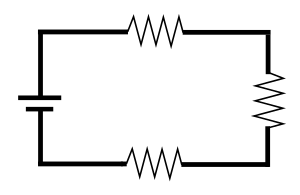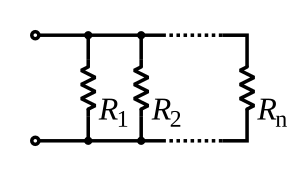# Series and parallel circuits facts for kids

Kids Encyclopedia Facts

Two types of electrical circuits are the series circuit, and the parallel circuit.

## Series CircuitThis is an example of a series circuit. In this picture, we see the current flowing from a power source, directly into three loads, and then back into the power source.

In a series circuit, the electrical current is only able to flow around a single path. The current will flow from a power source, such as a battery, into one or more electrical loads, such as a light bulb, and then back to the power source. In a series circuit, the same amount of amperage from the power source flows through each load. The Voltage in a series circuit is divided up across all of the loads. If one of the loads on a series circuit stops working, the current will not be able to flow through the rest of the circuit, and the rest of the loads will also stop working.

## Parallel circuitThis is an example of a parallel circuit. In this image, we see three resistors connected to a power source in parallel.

In a parallel circuit, the electrical current may flow along multiple paths before returning to the power source. The Voltage in a parallel circuit is the same across all of the loads in the circuit. In a parallel circuit, the amperage is divided up across all of the loads. If one of the loads in a parallel circuit stops working, the other loads in parallel will be able to continue to work."Potential difference is the same across all the component.In simpler terms identical bulbs connected in parallel will all be at the same brightness."-AlvinSeries and parallel circuits Facts for Kids. Kiddle Encyclopedia.